# 1709 Sometimes “Guess and Check” Is a Good Strategy

### Today’s Puzzle:

Most of the puzzles I publish are logic puzzles, and I encourage you to find the logic of the puzzle and not guess and check.  However, guess and check is a legitimate strategy in mathematics, and it is a legitimate strategy to solve this particular puzzle.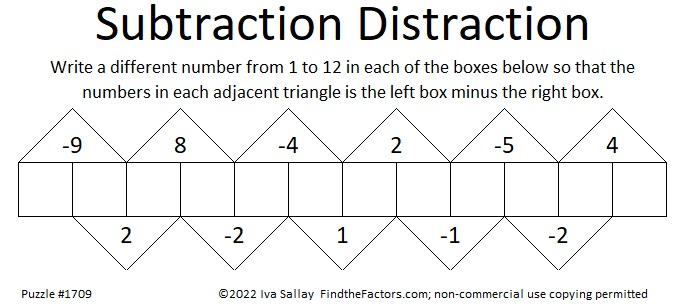Since one of the clues is -9, we know that the two boxes under it must be [1, 10], [2, 11], or [3, 12].

Suppose you assume it’s 1 – 10 = -9. If you fill out the rest of the boxes you would get: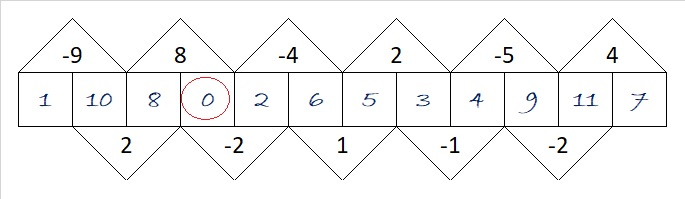You know that isn’t right because zero is not a number from 1 to 12. No problem. Simply add one to each of the numbers you wrote in, and the puzzle will be solved with only numbers from 1 to 12.

Suppose you assumed it’s 3 -12 = -9. The rest of the boxes would look like this:Again, 13 is not included in the numbers from 1 to 12, but you can fix it by subtracting 1 from each of the numbers you wrote in. Easy Peasy.

### Factors of 1709:

• 1709 is a prime number.
• Prime factorization: 1709 is prime.
• 1709 has no exponents greater than 1 in its prime factorization, so √1709 cannot be simplified.
• The exponent in the prime factorization is 1. Adding one to that exponent we get (1 + 1) = 2. Therefore 1709 has exactly 2 factors.
• The factors of 1709 are outlined with their factor pair partners in the graphic below.How do we know that 1709 is a prime number? If 1709 were not a prime number, then it would be divisible by at least one prime number less than or equal to √1709. Since 1709 cannot be divided evenly by 2, 3, 5, 7, 11, 13, 17, 19, 23, 29, 31, 37, or 41, we know that 1709 is a prime number.

### More About the Number 1709:

1709 is the sum of two squares:
35² + 22² = 1709.

1709 is the hypotenuse of a Pythagorean triple:
741-1540-1709, calculated from 35² – 22², 2(35)(22), 35² + 22².

Here’s another way we know that 1709 is a prime number: Since its last two digits divided by 4 leave a remainder of 1, and 35² + 22² = 1709 with 35 and 22 having no common prime factors, 1709 will be prime unless it is divisible by a prime number Pythagorean triple hypotenuse less than or equal to √1709. Since 1709 is not divisible by 5, 13, 17, 29, 37, or 41, we know that 1709 is a prime number.

# 1708 Happy Birthday, Jo Morgan!

### Today’s Puzzle:

A few days ago I published a new kind of factoring puzzle. Jo Morgan of Resourceaholic.com, keeps an eye out for new mathematical resources on Twitter. She was one of the first to notice and like my puzzle. Because of her, lots of other people noticed the puzzle, too. Today is Jo’s birthday, and I decided to make a similar puzzle for her to enjoy. You might find it slightly more difficult than the earlier puzzle, but use logic from the beginning, and you will be able to fit in all the factors.### Factors of 1708:

• 1708 is a composite number.
• Prime factorization: 1708 = 2 × 2 × 7 × 61, which can be written 1708 = 2² × 7 × 61.
• 1708 has at least one exponent greater than 1 in its prime factorization so √1708 can be simplified. Taking the factor pair from the factor pair table below with the largest square number factor, we get √1708 = (√4)(√427) = 2√427.
• The exponents in the prime factorization are 2, 1, and 1. Adding one to each exponent and multiplying we get (2 + 1)(1 + 1)(1 + 1) = 3 × 2 × 2 = 12. Therefore 1708 has exactly 12 factors.
• The factors of 1708 are outlined with their factor pair partners in the graphic below.### More About the Number 1708:

1708 is the hypotenuse of a Pythagorean triple:
308-1680-1708, which is 28 times (11-60-61).

# 1707 Subtraction Distraction

### Today’s Puzzle:

Even though this Subtraction Distraction puzzle has more boxes than the one I published a couple of weeks ago, it is actually an easier puzzle. Can you write the numbers 1 to 12 in the boxes so that each triangle is its adjacent left box minus its adjacent right box?### Factors of 1707:

1 + 7 + 0 + 7 = 15, a multiple of 3, so 1707 is divisible by 3.

• 1707 is a composite number.
• Prime factorization: 1707 = 3 × 569.
• 1707 has no exponents greater than 1 in its prime factorization, so √1707 cannot be simplified.
• The exponents in the prime factorization are 1 and 1. Adding one to each exponent and multiplying we get (1 + 1)(1 + 1) = 2 × 2 = 4. Therefore 1707 has exactly 4 factors.
• The factors of 1707 are outlined with their factor pair partners in the graphic below.### More About the Number 1707:

1707 is the hypotenuse of a Pythagorean triple:
693-1560-1707, which is 3 times (231-520-569).

# 1706 Can You Make the Factors Fit?

### Today’s Puzzle:

It’s 2022. Happy New Year! I wanted to make a puzzle that has 20 and 22 as clues and thought about what I could do.

I continue to be inspired by an old addition puzzle Sarah Carter @mathequalslove shared on Twitter:

I decided to tweak that puzzle into a multiplication puzzle. I ran into a problem, however. Having products in every triangle made the puzzle way too easy. How do I fix that? I removed some of the product clues. Can you use logic and factoring to know where each factor from 1 to 12 belongs? Can you determine the missing products? I hope you have lots of fun finding the puzzle’s only solution! And I hope you make the factors fit instead of having a fit trying!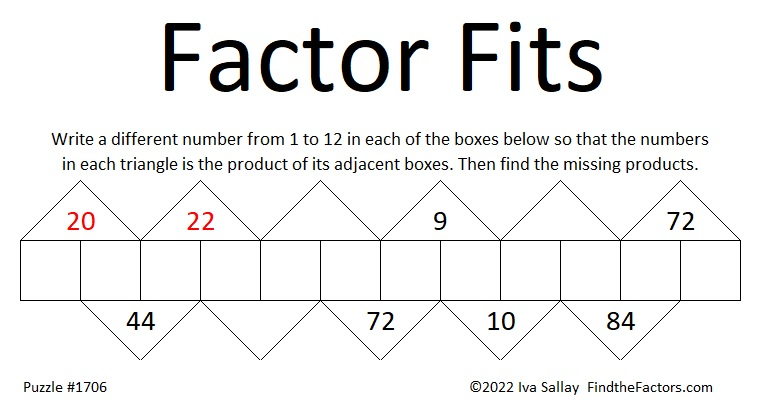Here’s something I haven’t told you before: I made lots of multiplication-table puzzles years before I started blogging. I wanted to give the puzzles a good name. At first, I called them “Turn the Tables on Multiplication” or “Turn the Tables” for short. I thought that title was clever but a little bit unwieldy. For a short time, I called the puzzles “Factor Fits.” It was a play on words because all the factors fit, but they might give you fits as you try to find them. I finally settled on “Find the Factors.” That title doubled as instructions for the puzzles. I still liked the name “Factor Fits,” and this puzzle lets me give new life to that name.

### Factors of 1706:

• 1706 is a composite number.
• Prime factorization: 1706 = 2 × 853.
• 1706 has no exponents greater than 1 in its prime factorization, so √1706 cannot be simplified.
• The exponents in the prime factorization are 1 and 1. Adding one to each exponent and multiplying we get (1 + 1)(1 + 1) = 2 × 2 = 4. Therefore 1706 has exactly 4 factors.
• The factors of 1706 are outlined with their factor pair partners in the graphic below.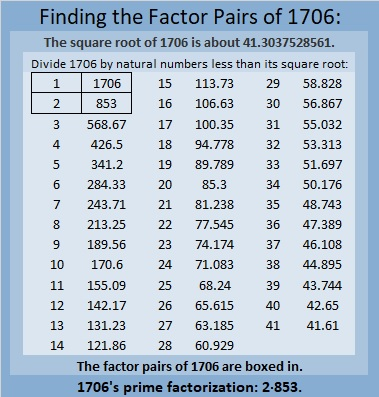### More About the Number 1706:

1706 is the sum of two squares:
41² + 5² = 1706.

1706 is the hypotenuse of a Pythagorean triple:
410-1656-1706, calculated from 2(41)(5), 41² – 5², 41² + 5².
It is also 2 times (205-828-853).

# 2022 Facts and Factors

### Today’s Puzzle:

Solve these two multiplication problems and see how mirror-like they are!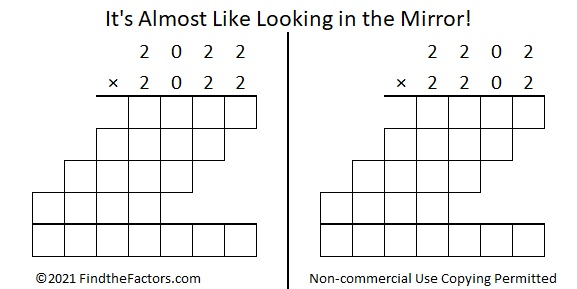Only 50 numbers less than 10000 can make a similar claim to fame:### Countdown to 2022:

Early in 2021, I found a countdown equation for 2022 that also involves its factors. A couple of weeks ago, I found another one. I can’t decide which one I like the best. Even though you can only focus on one equation at a time, the countdown will show both of them concurrently: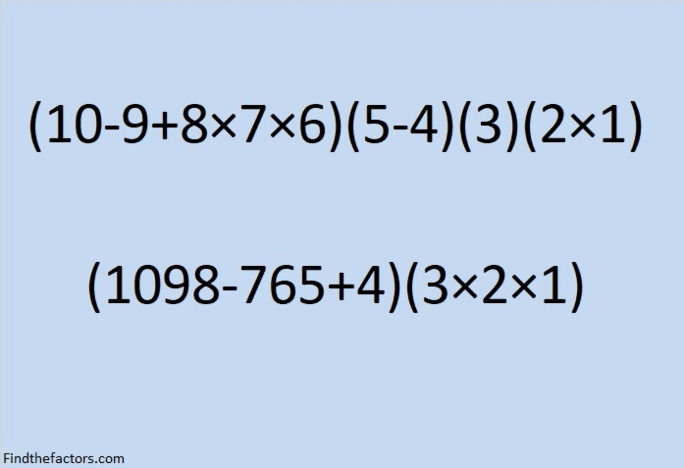make science GIFs like this at MakeaGif
Here is a still of the last frame:

### Factors of 2022:

You might be asked to find the factors of 2022 several times in the coming year.
It’s an even number, so it’s divisible by 2.
It’s also easy to remember that it is divisible by 3 because 2 + 2 + 2 = 6, a multiple of 3.
This graphic may help you remember that it is divisible by 337:
• 2022 is a composite number.
• Prime factorization: 2022 = 2 × 3 × 337.
• 2022 has no exponents greater than 1 in its prime factorization, so √2022 cannot be simplified.
• The exponents in the prime factorization are 1, 1, and 1. Adding one to each exponent and multiplying we get (1 + 1)(1 + 1)(1 + 1) = 2 × 2 × 2 = 8. Therefore 2022 has exactly 8 factors.
• The factors of 2022 are outlined with their factor pair partners in the graphic below.

### More About the Number 2022:

2022 is in the hypotenuse of a Pythagorean triple:
1050-1728-2022, which is 6 times (175-288-337).

It is also the short leg in three other Pythagorean triples:
2022-2696-3370, which is (3-4-5) times 674,
2022-340704-340710, which is 6 times (337-56784-56785), and
2022-1022120-1022122, which is 2 times (1011-511060-511061)

2022 uses only 2’s and 0’s in base 10 and in base 3:
2022₁₀ = 2202220₃.

2022 is the sum of positive consecutive numbers in three different ways: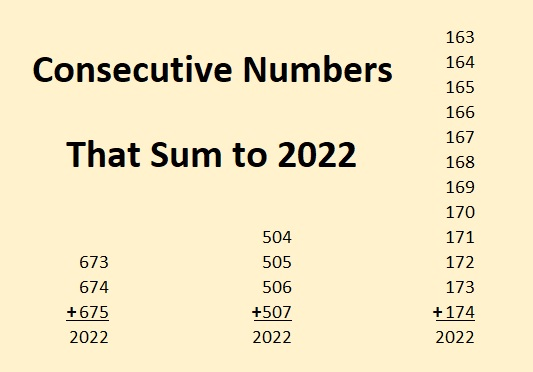### 2022 Magic Squares: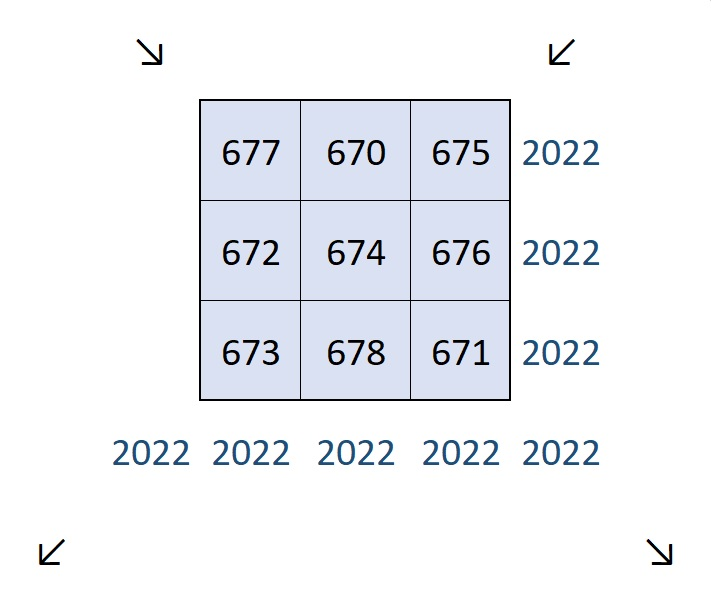All of the above are the facts that I came up with. Lots of people on Twitter have found other facts about 2022. Be sure to check them out!

### Tweets Celebrating 2022’s Mathematical Properties:

Here are some tweets about 2022 that I’ve seen on Twitter. I’ll add more as I see them. They are not listed in order of difficulty, but more or less, in the order that I saw them.

I’ll end with this thread that you’ll just have to click on to appreciate. It’s packed with tons of mathematical reasons 2022 will a fabulous year!

# 1705 The Seat Numbers from Jimmy Fallon’s Twelve Days of Christmas Sweaters

### Today’s Puzzle:

Jimmy Fallon’s Twelve Days of Christmas Sweaters tradition has become something I look forward to each December. The sweaters are one-of-a-kind masterpieces. I love when the sweaters are revealed. Jimmy reaches into a bright red Christmas stocking and randomly pulls out a number, the seat number of the winner of the sweater. Miraculously,  the winner of each sweater looks fabulous in it, no matter how big or small the winner is. I love this tradition, the sweaters, the winners modeling the sweaters, but I also love hearing the seat numbers. Each seat number has something special about it. (Just because it is a number!) By day three, I knew I wanted to blog about the numbers this year. The seat numbers were 295, 257, 314, 270, 419, 126, 256, 417, 433, 242, 232, and 120. I immediately knew something special about several of the numbers, but some of them I had to research. Can you figure out what is so special about each one?

Three of the seat numbers were primes. Which three?

One of those primes is both the fourth Fermat prime and the second-largest known Fermat prime. Which prime number is that?

Two of the numbers were palindromes (numbers that read the same forward and backward). Which two?

One of the seat numbers is equal to 1 × 2 × 3 × 4 × 5. Mathematicians write that as 5! Which seat number is equal to 5!?

One of the numbers is 10π rounded. Which one?

### How Do Some of the Seat Numbers Shape Up?

Two of the numbers were decagonal numbers. Which two?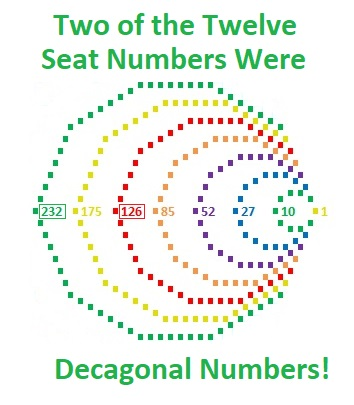126 is not only a decagonal number, but it is also a pyramid formed by stacking the first six pentagonal numbers on top of each other.
1 + 5 + 12 + 22 + 35 + 51 = 126.120 comes in THREE shapes.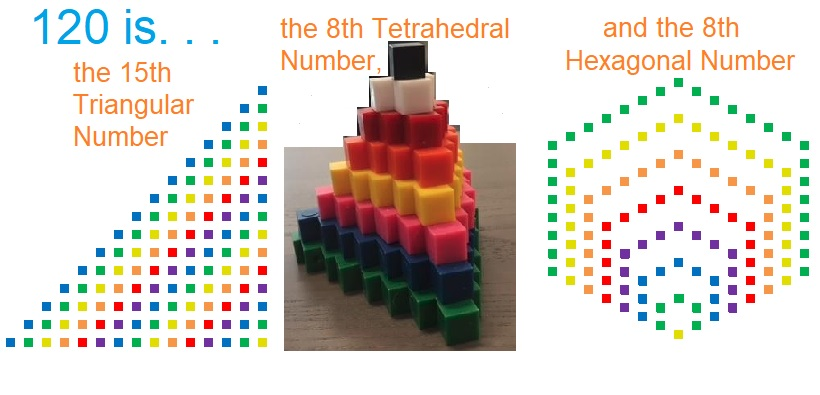One of the seat numbers is a star: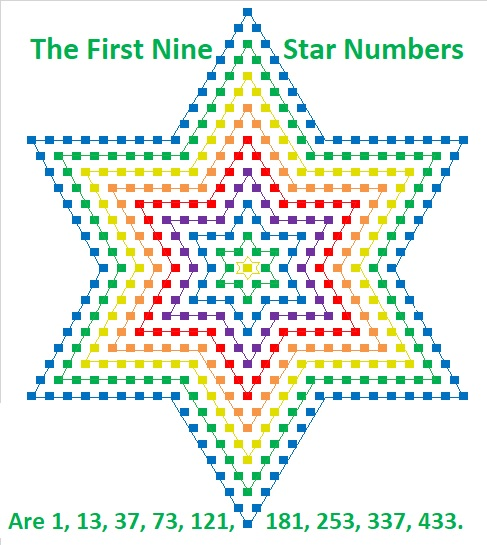### Something Special About Each Seat Number: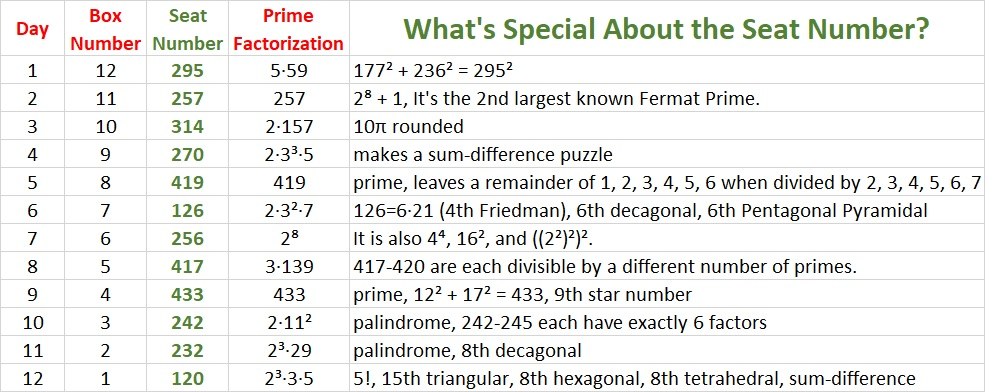I’ll explain some of these reasons below.

### Three of the Numbers Were the First Numbers to do Something Special:

242 is the smallest number whose square root can be simplified that is followed by three other numbers whose square root can also be simplified. Also, all four numbers have exactly six factors. Numbers with exactly six factors always have simplifiable square roots.

• 242 = 2·11²; its six factors are 1, 2, 11, 22, 121, 242.
• 243 = 3⁵; its six factors are 1, 3, 9, 27, 81, 243.
• 244 = 2²·61; its six factors are 1, 2, 4, 61, 122, 244.
• 245 = 7²·5; its six factors are 1, 5, 7, 35, 49, 245.417 is the smallest number that is the first of four consecutive integers that are divisible by a different number of primes.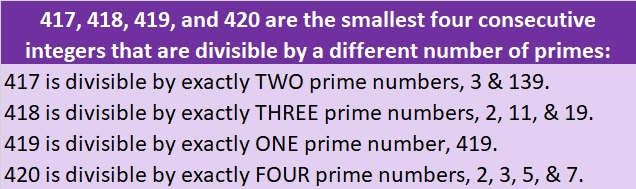419 is one less than 420, the smallest number divisible by 1, 2, 3, 4, 5, 6, and 7. As a consequence of that, 419 is the smallest number that leaves a remainder of 1, when it is divided by 2, a remainder of 2, when it is divided by 3, a remainder of 3, when it is divided by 4, a remainder of 4, when it is divided by 5, a remainder of 5, when it is divided by 6, and a remainder of 6, when it is divided by 7. This next graphic is a different way to make the same point.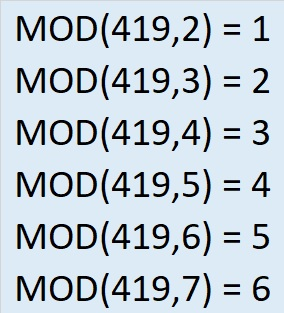### What Do I Mean by Sum-Difference?

Two of the seat numbers have factor pairs that make sum-difference: the numbers in one of its factor pairs add up to a particular number and the numbers in a different factor pair subtract to the same number. Coincidentally, both of the seat numbers are related to 30, another number that makes sum-difference.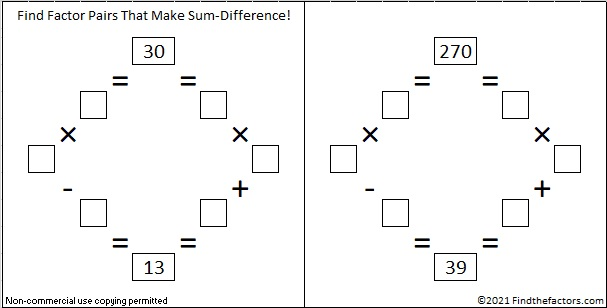I’ve so enjoyed discovering what made all those seat numbers special, and I hope that you have enjoyed reading about them as well!

Since this is my 1705th post, I’ll write a little about that number, as well.

### Factors of 1705:

• 1705 is a composite number.
• Prime factorization: 1705 = 5 × 11 × 31.
• 1705 has no exponents greater than 1 in its prime factorization, so √1705 cannot be simplified.
• The exponents in the prime factorization are 1, 1, and 1. Adding one to each exponent and multiplying we get (1 + 1)(1 + 1)(1 + 1) = 2 × 2 × 2 = 8. Therefore 1705 has exactly 8 factors.
• The factors of 1705 are outlined with their factor pair partners in the graphic below.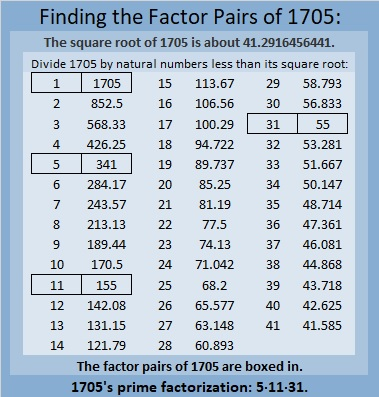### More About the Number 1705:

1705 is the hypotenuse of a Pythagorean triple:
1023 1364 1705, which is (3-4-5) times 341.

# 1704 Christmas Factor Tree

### Today’s Puzzle:

If you know the factors of the clues in this Christmas tree, and you use logic, it is possible to write each number from 1 to 12 in both the first column and the top row to make a multiplication table. It’s a level six puzzle, so it won’t be easy, even for adults, but can YOU do it?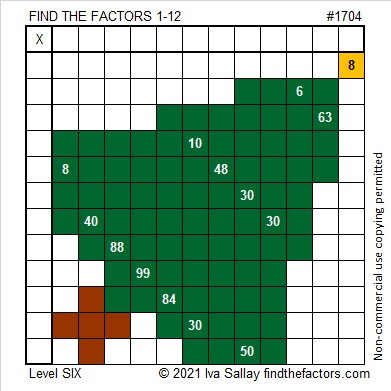### Factors of 1704:

If you were expecting to see a factor tree for the number 1704, here is one of several possibilities: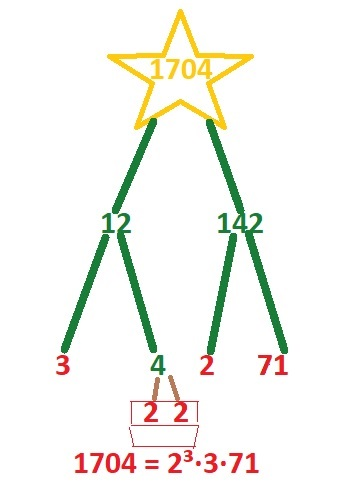• 1704 is a composite number.
• Prime factorization: 1704 = 2 × 2 × 2 × 3 × 71, which can be written 1704 = 2³ × 3 × 71.
• 1704 has at least one exponent greater than 1 in its prime factorization so √1704 can be simplified. Taking the factor pair from the factor pair table below with the largest square number factor, we get √1704 = (√4)(√426) = 2√426.
• The exponents in the prime factorization are 3,1 and 1. Adding one to each exponent and multiplying we get (3 + 1)(1 + 1) (1 + 1) = 4 × 2 × 2 = 16. Therefore 1704 has exactly 16 factors.
• The factors of 1704 are outlined with their factor pair partners in the graphic below.### More About the Number 1704:

1704 is the difference of two squares in FOUR different ways:
427² – 425² = 1704,
215² – 211² = 1704,
145² – 139² = 1704, and
77² – 65² = 1704.

Why was Six afraid of Seven? Because Seven ate Nine.
1704 is 789 in a different base:
1704₁₀ = 789₁₅ because 7(15²) + 8(15¹) + 9(15º) = 1704.

# 1703 A Wreath to Hang on Your Door

### Today’s Puzzle:

A wreath is a lovely decoration to hang on your door at Christmastime. This one might have a few thorns in it, but if you are careful, they won’t bother you in the least. Just use logic to write the numbers 1 to 12 in both the first column and the top row so that those numbers and the given clues create a multiplication table.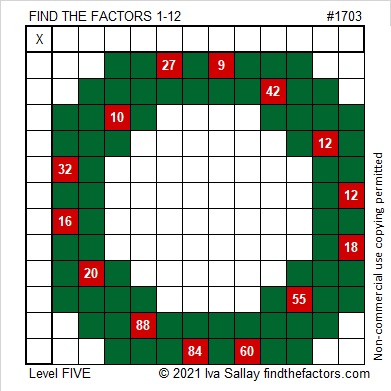### Factors of 1703:

• 1703 is a composite number.
• Prime factorization: 1703 = 13 × 131.
• 1703 has no exponents greater than 1 in its prime factorization, so √1703 cannot be simplified.
• The exponents in the prime factorization are 1 and 1. Adding one to each exponent and multiplying we get (1 + 1)(1 + 1) = 2 × 2 = 4. Therefore 1703 has exactly 4 factors.
• The factors of 1703 are outlined with their factor pair partners in the graphic below.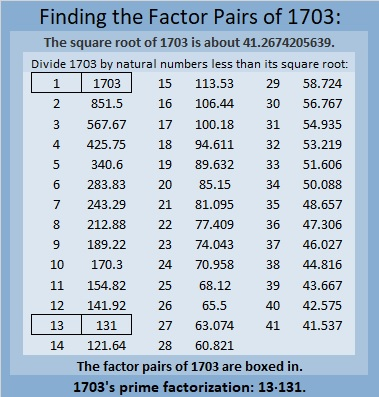### More About the Number 1703:

Did you notice a cool pattern in 1703’s prime factorization?
13·131 =1703.

1703 is the hypotenuse of a Pythagorean triple:
655-1572-1703, which is (5-12-13) times 131.

1703 is the difference of two squares in two different ways:
852² – 851² = 1703, and
72² – 59² = 1703.

# 1702 A Puzzle Idea from @mathequalslove Tweaked into a Subtraction Puzzle That Directs You to a Post from NebusResearch

### Today’s Puzzle:

Joseph Nebus is nearly finished with all the posts in his Little 2021 Mathematics A to Z series. Every year he requests that his readers give him mathematical subjects to write about. At my suggestion, he recently wrote about subtraction, and how it is a subject that isn’t always as elementary as you might expect.  With a touch of humor, we learn that subtraction opens up whole new topics in mathematics.

I wanted to make a puzzle to commemorate his post. I gave it some thought and remembered a tweet from Sarah Carter @mathequalslove:

That puzzle originated from The Little Giant Encylopedia of Puzzles by the Diagram Group. I wondered how the puzzle would work if it were a subtraction puzzle instead of an addition puzzle, and here’s how I tweaked it: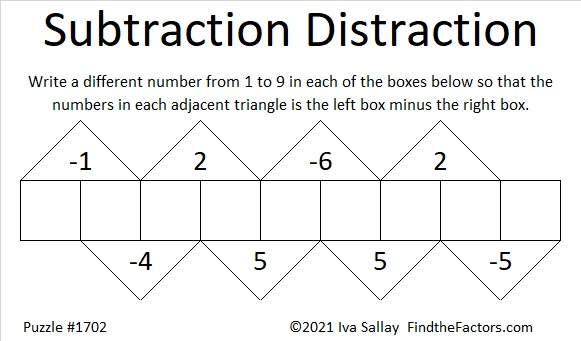There is only one solution. I hope you will try to find it! If you would like a hint, I’ll share one at the end of this post.

### Factors of 1702:

• 1702 is a composite number.
• Prime factorization: 1702 = 2 × 23 × 37.
• 1702 has no exponents greater than 1 in its prime factorization, so √1702 cannot be simplified.
• The exponents in the prime factorization are 1, 1, and 1. Adding one to each exponent and multiplying we get (1 + 1)(1 + 1)(1 + 1) = 2 × 2 × 2 = 8. Therefore 1702 has exactly 8 factors.
• The factors of 1702 are outlined with their factor pair partners in the graphic below.### More About the Number 1702:

1702 is the hypotenuse of a Pythagorean triple:
552-1610-1702, which is (12-35-37) times 46.

1702² = 2896804, and
2197² = 4826809.
Do you notice what OEIS.org noticed about those two square numbers?

### Puzzle Hint:

Here’s how I solved the puzzle: I let the rightmost box be x. Then using the values in the adjacent triangles and working from right to left, I wrote the values of the other boxes in terms of x.x – 5 went in the box that is second to the right,
x – 5 + 2 = x – 3 went in the next box,
x – 3 + 5 = x + 2,
x + 2 – 6 = x – 4,
x – 4 + 5 = x + 1, and so on until I had assigned a value in terms of x for every box.

Think about it, and this hint should be enough for you to figure out where the numbers from 1 to 9 need to go.

# 1701 Is a Decagonal Number

### Today’s Puzzle:

There is a pattern to the decagonal numbers. Can you figure out what it is?### Factors of 1701:

1701 is divisible by nine because 1 + 7 + 0 + 1 = 9.

• 1701 is a composite number.
• Prime factorization: 1701 = 3 × 3 × 3 × 3 × 3 × 7, which can be written 1701 = 3⁵ × 7.
• 1701 has at least one exponent greater than 1 in its prime factorization so √1701 can be simplified. Taking the factor pair from the factor pair table below with the largest square number factor, we get √1701 = (√81)(√21) = 9√21.
• The exponents in the prime factorization are 5 and 1. Adding one to each exponent and multiplying we get (5 + 1)(1 + 1) = 6 × 2 = 12. Therefore 1701 has exactly 12 factors.
• The factors of 1701 are outlined with their factor pair partners in the graphic below.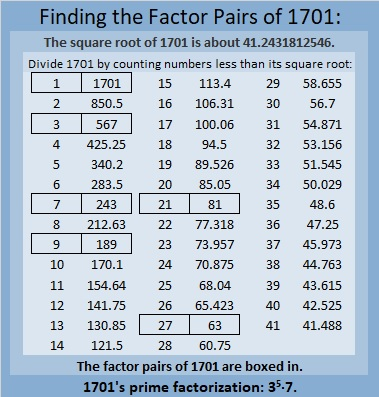### More About the Number 1701:

1701 is the difference of two squares in SIX different ways.
851² – 850² = 1701,
285² – 282² = 1701,
125² – 118² = 1701,
99² – 90² = 1701,
51² – 30² = 1701, and
45² – 18² = 1701.

1701 is the 21st decagonal number because
21(4·21 – 3) =
21(84-3) =
21(81) = 1701.

There is decagonal number generating function:
x(7x+1)/(1-x)³ = x + 10x² + 27x³ + 52x⁴ + 85x⁵ + . . .

The 21st term of that function is 1701 x²¹.